## Equivalence Class

An equivalence class is defined as a Subset of the form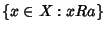, whereis an element of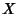and the Notation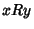'' is used to mean that there is an Equivalence Relation betweenand. It can be shown that any two equivalence classes are either equal or disjoint, hence the collection of equivalence classes forms a partition of. For all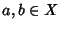, we have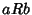Iffand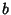belong to the same equivalence class.

A set of Class Representatives is a Subset ofwhich contains Exactly One element from each equivalence class.

Fora Positive Integer, and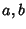Integers, consider the Congruence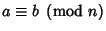, then the equivalence classes are the sets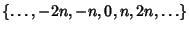,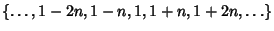etc. The standard Class Representatives are taken to be 0, 1, 2, ...,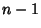.